# Equivalence Of F.S.A (Finite State Automata)

• Last Updated : 01 Jul, 2021

An Automaton is a machine that has a finite number of states. Any Two Automaton is said to be equivalent if both accept exactly the same set of input strings.
Two Automaton are equivalent if they satisfy the following conditions :

1. The initial and final states of both the automatons must be same.
2. Every pair of states chosen is from a different automaton only.
3. While combining the states with the input alphabets, the pair results must be either both final states or intermediate states.(i.e both should lie either in the final state or in the non-final state).
4. If the resultant pair has different types of states, then it will be non-equivalent. (i.e. One lies in the final state and the other lies in the intermediate state ).

Example 1 –
Consider Two Different Automaton shown below in Figure 1.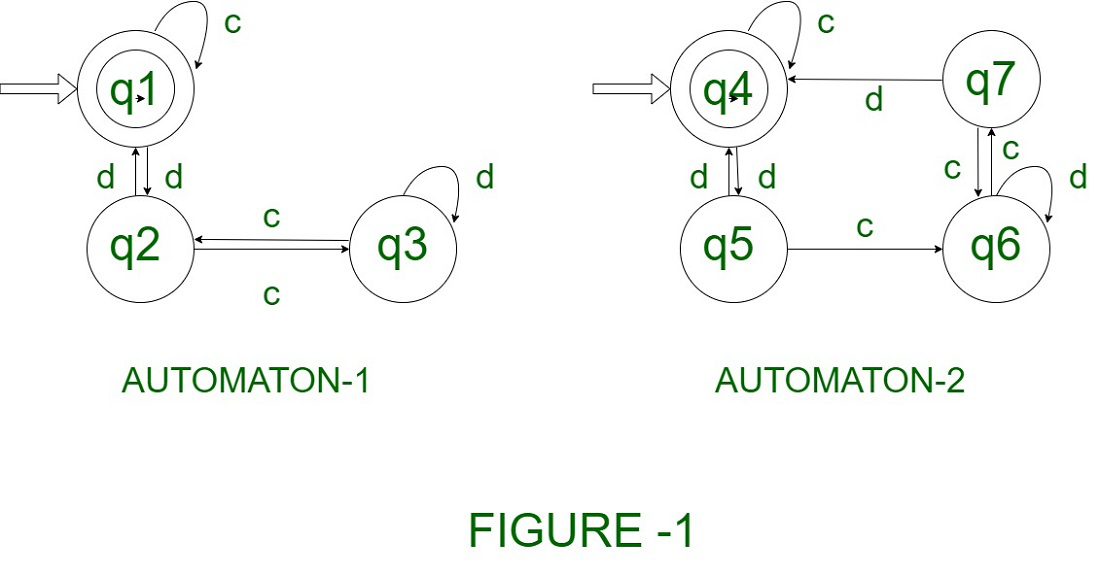Solution –
Step 1 – Since the initial and final states of both the automaton are the same, so it verifies.
Step 2 – Check for each state by making a table of states with respect to input alphabets.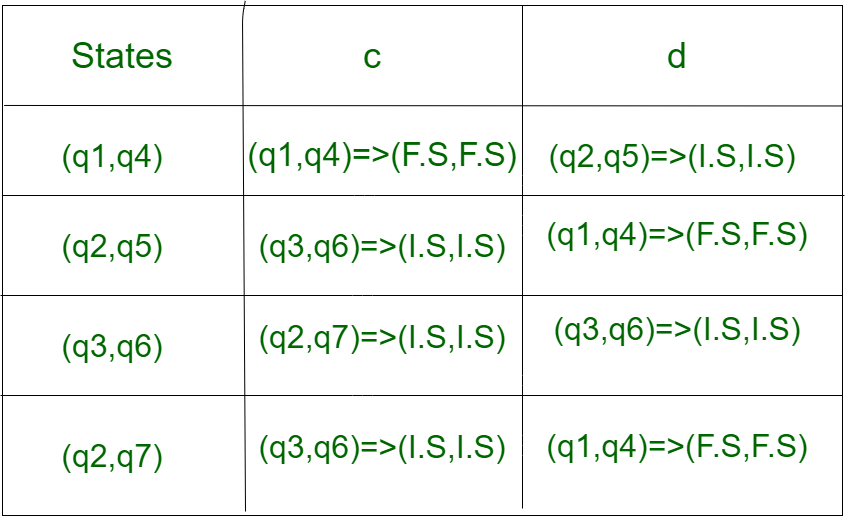Here ,
F.S represents -> Final State.
I.S represents -> Intermediate State (Non-Final State) .

Step 3 – For every pair of states, the resultant states lie either in F.S or in I.S as a combination.

Conclusion – Both the Automaton are equivalent.

Note : If the resultant pair of states has a different combination of states(i.e. either (F.S, I.S) or (I.S, F.S), then both the automata are said to be non-equivalent.

Example 2 –
Consider Two Different Automaton shown below in Figure 2.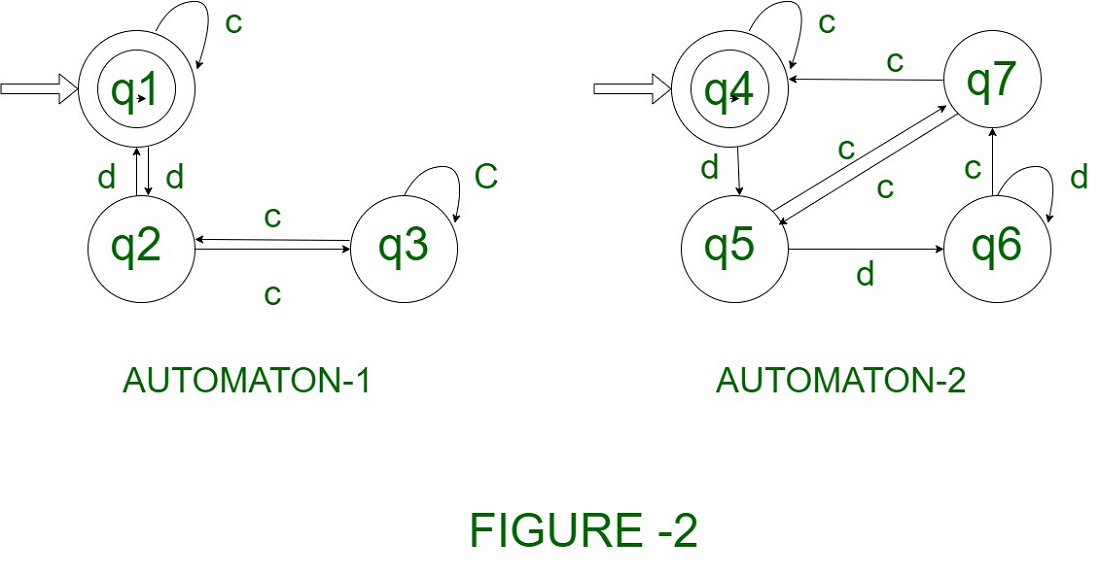Solution –
Step 1 – Since the initial and final states of both the automaton are the same so it verifies.
Step 2 – Check for each state by making a table of states with respect to input alphabets.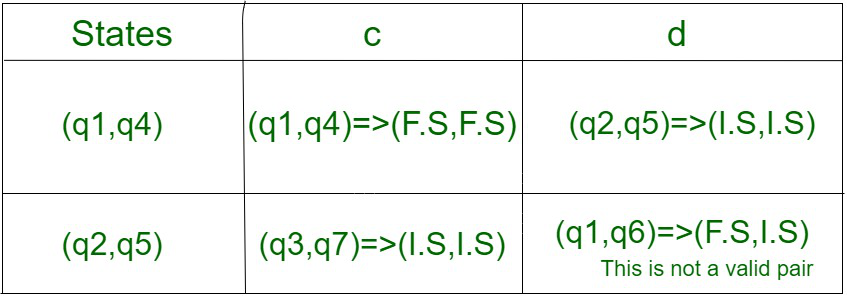Here ,
F.S represents -> Final State.
I.S represents -> Intermediate State (Non-Final State) .
Step 3 – For the first pair of states, the resultant states lie in F.S as a combination
Step 4 – But for the pair (q2,q5) when operated over input alphabets they lie in different states. (i.e. one in F.S and the other in I.S).

Conclusion – The Given Automata are not equivalent.

Example 3 –
Consider Two Different Automaton shown below in Figure 3.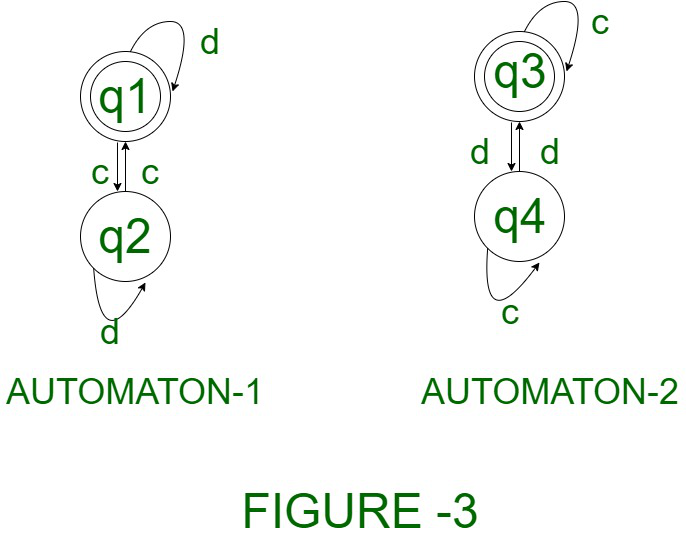Solution –
Step 1 – Since the initial and final states of both the automaton are the same so it verifies.
Step 2 – Check for each state by making a table of states with respect to input alphabets.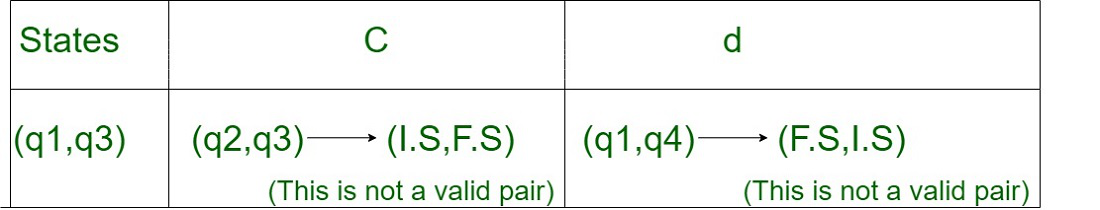Here,
F.S represents -> Final State.
I.S represents -> Intermediate State (Non-Final State) .
Step 3 – For the pair (q1,q3), when operated over input alphabets they lie in different states. (i.e. one in F.S and the other in I.S).

Conclusion – The Given Automata are not equivalent.

Example 4 –
Consider Two Different Automaton shown below in Figure 4.

Solution –
Step 1 – Since the initial and final states of both the automaton are the same, so it verifies.
Step 2 – Check for each state by making a table of states with respect to input alphabets.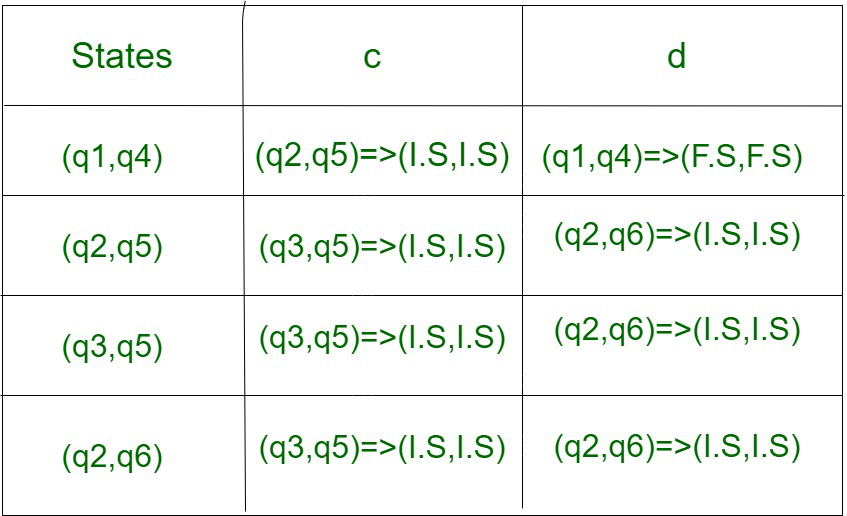Here,
F.S represents -> Final State.
I.S represents -> Intermediate State (Non-Final State) .
Step 3 – For every pair of states, the resultant states lie either in F.S or in I.S as a combination.

Conclusion – Both the Automaton are equivalent.

My Personal Notes arrow_drop_up